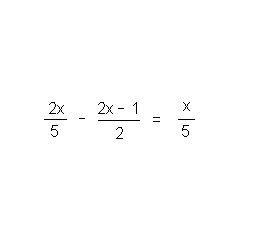# Thread: Help with an equation!

1. ## Help with an equation!

I need help solving the equation below!!! What are the steps to solving it?2.Originally Posted by AshI need help solving the equation below!!! What are the steps to solving it?$\displaystyle \frac{2x}{5} - \frac{2x - 1}{2} = \frac{x}{5}$

Most people hate fractions, so let's get rid of these first. We need to find a common denominator, and I would choose 2 * 5 = 10. So I'm going to multiply both sides of the equation by 10:
$\displaystyle 10 \cdot \left ( \frac{2x}{5} - \frac{2x - 1}{2} \right ) = 10 \cdot \left ( \frac{x}{5} \right )$

$\displaystyle 2 \cdot (2x) - 5 \cdot (2x - 1) = 2 \cdot (x)$

$\displaystyle 4x - 10x + 5 = 2x$

$\displaystyle -6x + 5 = 2x$

$\displaystyle -2x - 6x + 5 = -2x + 2x$

$\displaystyle -8x + 5 = 0$

$\displaystyle -8x + 5 - 5 = 0 - 5$

$\displaystyle -8x = -5$

$\displaystyle \frac{-8x}{-8} = \frac{-5}{-8}$

$\displaystyle x = \frac{5}{8}$

-Dan

3.Originally Posted by AshI need help solving the equation below!!! What are the steps to solving it?(2x)/5 - (2x - 1)/2 = x/5

Multiply each term by 10;

10*[2x/5] - 10*[(2x - 1)/2] = 10*[x/5]

2*(2x) - 5*(2x - 1) = 2x

4x - 10x + 5 = 2x

-6x + 5 = 2x

5 = 8x

5/8 = x

Check: plug it in and you will see that

1/8 = 1/8

#### Search Tags

equation